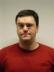## ()

\$ perl -Mstrict -we'\$_ = time; print "\$_\n"'
1583887020

<.<

\$ perl -Mstrict -we'\$_ = CORE::time; print "\$_\n"'
1583887039

>.>

\$ perl -Mstrict -we'\$_ = time - CORE::time; print "\$_\n"'
0

-_- ...

\$ perl -Mstrict -we'BEGIN{*CORE::GLOBAL::time=sub{42}} \$_ = time - CORE::time; print "\$_\n"'
42

(┛◉Д◉)┛彡┻━

\$ perl -Mstrict -we'BEGIN{*CORE::GLOBAL::time=sub{42}} \$_ = time() - CORE::time; print "\$_\n"'
-1583886770

🧑🔬

\$ alias deparse='perl -Mstrict -MO=Deparse,-p -we'

\$ deparse 'BEGIN{*CORE::GLOBAL::time=sub{42}} \$_ = time - CORE::time; print "\$_\n"'
BEGIN { \$^W = 1; }
use strict;
sub BEGIN {
(*CORE::GLOBAL::time = sub {
42;
}
);
}
(\$_ = time((-time))); # !!!
print("\$_\n");
-e syntax OK

\$ deparse '\$_ = time - CORE::time; print "\$_\n"'
BEGIN { \$^W = 1; }
use strict;
(\$_ = (time - time)); # O.o
print("\$_\n");
-e syntax OK

\$ deparse 'BEGIN{*CORE::GLOBAL::time=sub{42}} \$_ = time() - CORE::time; print "\$_\n"'
BEGIN { \$^W = 1; }
use strict;
sub BEGIN {
(*CORE::GLOBAL::time = sub {
42;
}
);
}
(\$_ = (time() - time)); # ✓
print("\$_\n");
-e syntax OK

🤔

\$ perl -Mstrict -we'sub foo{42} \$_ = foo - CORE::time; print "\$_\n"'
42

\$ perl -Mstrict -we'sub foo{42} \$_ = foo() - CORE::time; print "\$_\n"'
-1583887692

🧑💡

\$ perl -Mstrict -we'sub foo{42} print prototype(\&foo), \$/'
Use of uninitialized value in print at -e line 1.

\$ perl -Mstrict -we'BEGIN{*CORE::GLOBAL::time=sub{42}} print prototype(\&time) . "\n"'
Use of uninitialized value in concatenation (.) or string at -e line 1.

\$ perl -Mstrict -we'BEGIN{*CORE::GLOBAL::time=sub{42}} print ">" . prototype(\&CORE::time) . "<\n"'
><

()!

\$ perl -Mstrict -we'sub foo(){42} \$_ = foo - CORE::time; print "\$_\n"'
-1583888475

\$ perl -Mstrict -we'BEGIN{*CORE::GLOBAL::time=sub(){42}} \$_ = time - CORE::time; print "\$_\n"'
-1583888509

¯\_(ツ)_/¯I blog about Perl.